# Community

Blog Events Webinars Tutorials Forum
×
Community Blog How to Implement Neural Networks with TensorFlow

# How to Implement Neural Networks with TensorFlow

Neural networks and TensorFlow solve many complicated real world problems in a simplified manner. This article explains the same with Python coding, which is very popular because of deep learning.Deep Learning and Neural Networks are currently two of the hottest topics in the field of artificial intelligence (AI). Both deep learning and neural networks are necessary to make sense out of large amounts of data, especially when it comes to Big Data applications.

### When to Use Neural Networks?

The "deeper" version of neural networks is making breakthroughs in many fields such as image recognition, speech, and natural language processing.

But what is more important to understand is the application of neural networks. You need to keep the following in mind:

#### 1.Neural networks require a great deal of understanding

Think of a neural network as a kid. . He observes how his parents walk first, and then he walks independently. In each step, he learns how to carry out a specific task. If you don't let him walk, he may never learn how to walk. The more "data" you give him, the better is the effect.

#### 2.Right type of neural network for your problem

Every single problem has its own difficulty. Data determines how you solve the problem. For example, if the problem is about generation of sequences, a recurrent neural network would be more appropriate, but if it is about images, you may need a convolutional neural network instead.

#### 3.Hardware requirements are crucial to run a deep neural network model

Neural networks were "discovered" long ago, but they only got popular in recent years, thanks to the growth of computing power. If you want to solve real-world problems with those networks, be prepared to buy some pieces of high-performance hardware.

### How to Solve Problems with Neural Networks

A neural network is a special type of machine learning (ML) algorithm. Just like any other ML algorithm, neural networks follow the conventional ML workflows including data preprocessing, model building, and model evaluation. Here is a step by step guide that I have created to solve problems with neural networks:

•Conduct a survey to see which neural network architecture is most suitable for the problem to be solved.

•Define the neural network architecture with your selected language/library.

•Convert your data into the correct format and break it into batches.

•Preprocess the data based on your needs.

•Add more data to increase the data volume and make a better training model.

•Send the data batches into the neural network.

•Train and monitor training sets, and validate the changes in the data sets.

•Test your model and save it for future use.

In this article, I will focus on image data. Let's learn about images before we study TensorFlow.

Images are mostly arranged in 3D arrays, and the dimensions refer to the height, width and color channels. For example, if you take a screenshot of your desktop now, the screenshot will be converted to a 3D array first and then compressed into .png or .jpg format.

Although images are very easy for humans to understand, they are hard for computers. This is called the semantic gap. Human brains can view images and understand the complete picture in seconds. However, computers see images as a set of numbers.

In earlier days, people tried to decompose images into "understandable" formats like "templates." For example, different faces always have a specific structure that is common to everyone, such as the positions of eyes and noses or the shapes of faces. However, this approach is not feasible as such "templates" don't hold when the number of objects to be recognized increases.

In 2012, deep neural network architecture won the ImageNet challenge, a major competition for identifying objects from natural scenes.

So which libraries/languages are usually used to solve image recognition problems? A recent survey reveals that the most popular deep learning library is Python's API, followed by those of Lua, Java and Matlab. Other popular libraries include:

•Caffe

•DeepLearning4j

•TensorFlow

•Theano

•Torch

Let's take a look at TensorFlow and some of its features and benefits:

TensorFlow is an open source software library that uses data flow graphs for numeric computation. The nodes in the graphs represent mathematical operations, while the edges represent the multidimensional data arrays (aka tensors) that are passed between them. The flexible architecture allows you to deploy the computation to one or more CPUs or GPUs in your desktops, servers, or mobile devices using a single API.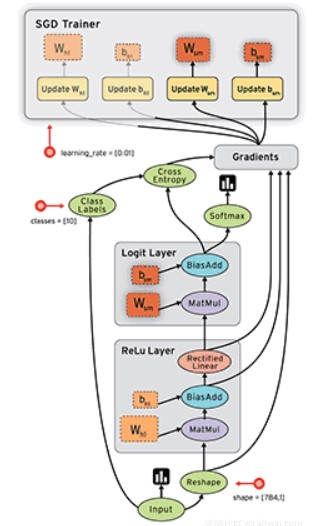TensorFlow is a piece of cake, if you have ever used NumPy. One of the main differences between the two is that TensorFlow follows a "lazy" programming paradigm. It first builds all the action graphs to be completed, then it "runs" the graphs when a "session" is invoked. Building a computation graph can be considered the major component of TensorFlow.

TensorFlow is more than just a powerful neural network library. It enables you to build other machine learning algorithms on it, such as decision trees or k-nearest-neighbors.

•An intuitive structure, because, as its name suggests, it has a "tensor flow" That allows you to easily see every part of the graph.

•Scope to easily perform distributed computation on the CPU/GPU.

•Platform flexibility to run the model anywhere on mobile devices, servers or Pcs.

### The Typical "Tensor Flow"

Every library has its own "implementation details", i.e. a method written in its encoding mode. For example, when executing scikit-learn, we first create the object of the desired algorithm, and then build a model on the training set and predict the test set. Example: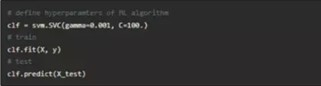As I said earlier, TensorFlow follows a "lazy" approach. The normal workflow of running a program in TensorFlow is as follows:

•Create a computation graph on any math operation of your choice that is supported by TensorFlow.

•Initialize variables.

•Create a session.

•Run the graph in the session.

•Close the session.

Next, let's write a small program to add two numbers!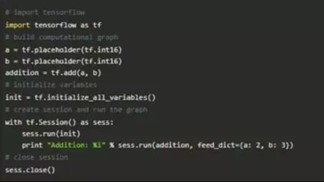### Implementing Neural Networks in TensorFlow

Note: We can use different neural network architectures to solve this problem, but for the sake of simplicity, we need to implement feedforward multilayer perceptrons.

The common implementation of neural networks is as below:

•Define the neural network architecture to be compiled.

•Transfer the data to your model.

•Divide the data into batches and then preprocess them.

•Add the batches to the neural network for training.

•Display the accuracy of specific time steps.

•Save the model for future use after training.

•Test the model on new data and check its implementation.

Our challenge here is to identify the numbers in given 28x28 images. We will take some images for training, and the remaining will be used to test our model. So, we first download the data set that contains the compressed files of all the images in the data set: train.csv and test.csv. The data set doesn't provide any additional functions, instead, it just contains the original images in .png format.

Now, we will use TensorFlow to build a neural network model. For this, you should first install TensorFlow on your system.

We will follow the steps as described in the template above. Create a Jupyter notebook with Python 2.7 kernel and follow the steps below.

Import all the required modules: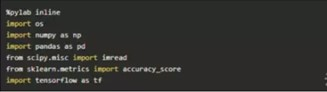Set the initial value so that we can control the randomness of the model: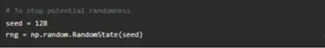Set the directory path to save the data: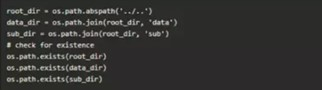Let's look at the data set. The data files are in .csv format and have corresponding tags in their filenames: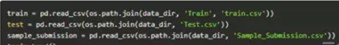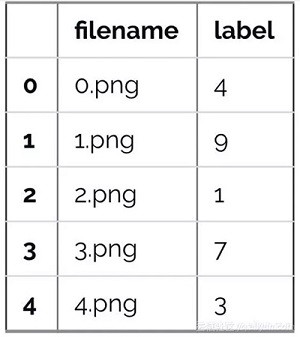Let's check out what our data looks like!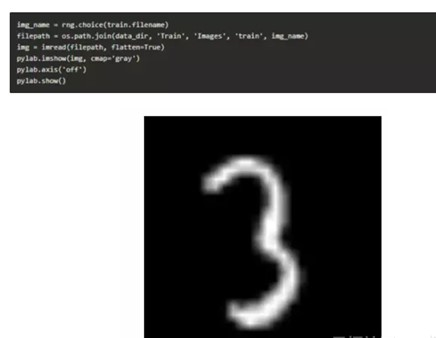The image above is represented as a NumPy array as below: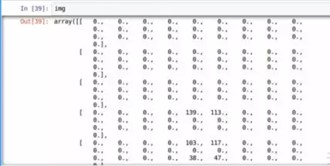For simpler data processing, let's store all the images as NumPy arrays: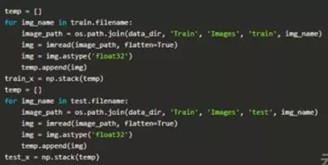Since this is a typical ML problem, we need to create a validation set in order to test the functionality of our model.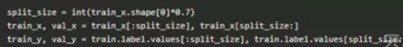Now, let's define some helper functions for later use: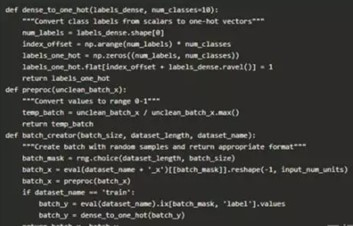Let's define our neural network architecture. We define a neural network with three layers, namely, input layer, hidden layer, and output layer. The numbers of neurons in the input and the output are fixed because the input is 28x28 images and the output is 10x1 vectors. We have 500 neurons in the hidden layer. This number can vary according to your needs.

0 0 0
Share on

# Related Products

• ##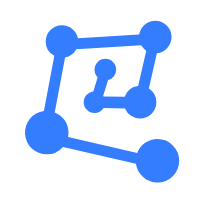Machine Learning Platform For AI

An end-to-end platform that provides various machine learning algorithms to meet your data mining and analysis requirements.

• ##Online Education Solution

This solution enables you to rapidly build cost-effective platforms to bring the best education to the world anytime and anywhere.

• ##Epidemic Prediction Solution

This technology can be used to predict the spread of COVID-19 and help decision makers evaluate the impact of various prevention and control measures on the development of the epidemic.

• ##Accelerated Global Networking Solution for Distance Learning

Alibaba Cloud offers an accelerated global networking solution that makes distance learning just the same as in-class teaching.